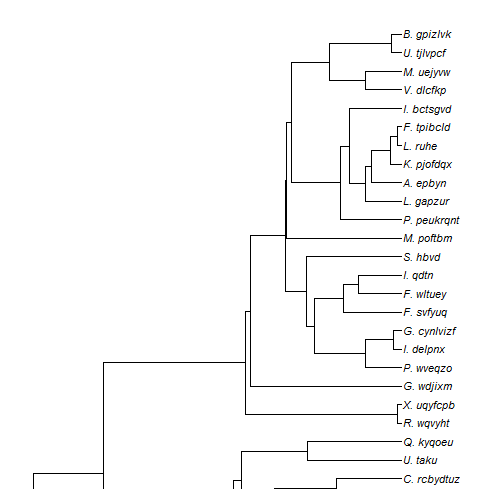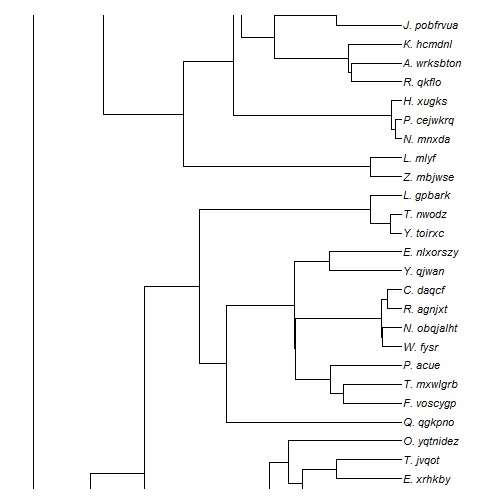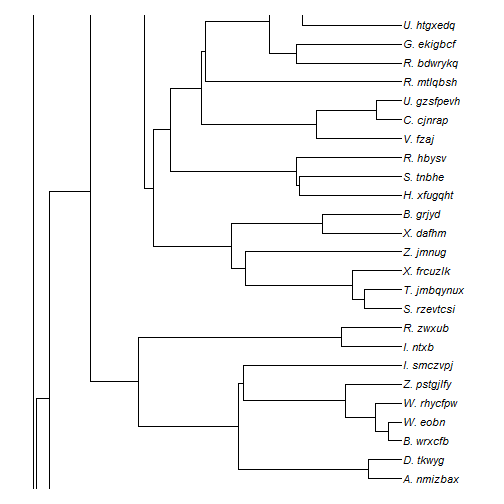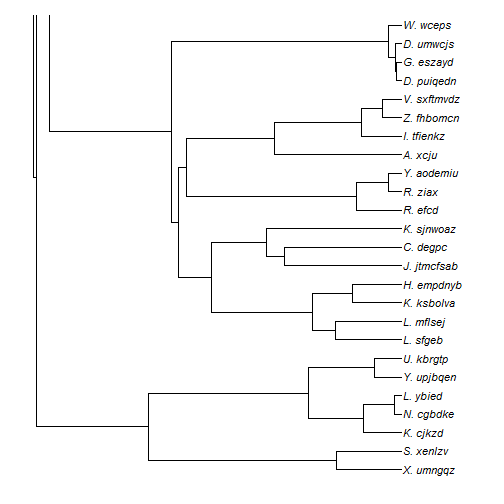## Thursday, March 5, 2015

### Splitting a tree over mutiple plotting devices or pages

An R-sig-phylo subscriber asked the following yesterday:

I'm searching for a way to plot a huge phylogenetic tree to multiple pages in one searchable pdf file from R…. Does anybody know if this is possible and an effective way of doing this?

Well, my first response was that phytools has a function, `splitplotTree`, that can be used to split a plotted tree across columns in a single plotting window, or across plotting devices. Consequently, I responded:

One option is to use the function `splitplotTree` in phytools. By default it plots the split tree in two columns, but there is an option to split it into two windows. Then each plotted tree could be saved as a PDF.

On further thought, I realized that there is a better way. `plotSimmap` in phytools (and, consequently, `plotTree`, which uses `plotTree`, which uses `plotSimmap` internally) allows the user to control the y-limits of the plot. As a result it would be straightforward to write a simple wrapper that split the tree using a moving window over the plotting area. It also allows us to relatively easily split our tree over more than two pages. The following is an example of what that might look like:

``````split.plotTree<-function(tree,splits=NULL,file=NULL,...){
ef<-0.037037037037
if(!is.null(file)) pdf(file,width=8.5,height=11)
if(is.null(splits)) splits<-(floor(0.5*Ntip(tree))+0.5)/Ntip(tree)
S<-matrix(c(0,splits,splits,1+1/Ntip(tree)),length(splits)+1,2)
S<-cbind(S[,1]+ef*(S[,2]-S[,1]),S[,2]-ef*(S[,2]-S[,1]))
for(i in nrow(S):1){
plotTree(tree,ylim=Ntip(tree)*S[i,],...)
}
if(!is.null(file)) oo<-dev.off()
}
``````

Note that in order to avoid having the tips & edges near the bottom of each page plot at the top of the following page, I had to trim ~4% off of the top & bottom of each plot. This is because for a given y range, R will create a plotting area that is 4% on each size larger than the area specified.

OK, now let's try it:

``````library(phytools)
n<-100
## create realistic tip labels
foo<-function(i) paste(sample(LETTERS,1),"._",paste(sample(letters,round(runif(1,min=4,max=8))),
collapse=""),sep="")
tree<-pbtree(n=n,tip.label=sapply(1:n,foo))
splits<-c(0.255,0.505,0.755)
split.plotTree(tree,splits,ftype="i",mar=rep(1.1,4),fsize=0.9,lwd=1)
``````The splits mark the proportions of the plotted graph that I want to be displayed on each page.

We can also send the output to a multi-page PDF:

``````split.plotTree(tree,splits,ftype="i",mar=rep(1,4),file="split.plotTree.pdf",lwd=1)
``````

The output is here: split.plotTree.pdf.

That's it.

1.Hi Liam,

This post is really helpful. I have a slight problem with my tree though. I used make.simmap to make an ancrestal reconstruction tree for discrete characters. The color coding is red for absent and blue for present. When I am using the above wrapper, the colors are not appearing on the final split tree. I tried changing the wrapper to include "cols", but in vain. Could you please help me with this?

2.Hi Liam, I've got a similar problem. I would like to use the splitplotTree to cut a huge tree into smaller sections in order to properly visualize the results of an ancestral state reconstruction.

I am using this code for the normal tree:

plot(tree, show.node.label=FALSE, show.tip.label = FALSE, label.offset=3,cex=0.4)

tiplabels(pie = Trait_matrix[tree\$tip.label, ], piecol = c("red","green"), cex = 0.50)

nodelabels(pie=Trait_ARD_model\$lik.anc, piecol=c("red","green"), cex = 0.50)

Can I (and if so how) combine this with the splitplotTree function?

3.Thanks this post! Is there a way to use this in conjunction with the plotTree.barplot function?

Note: due to the very large amount of spam, all comments are now automatically submitted for moderation.